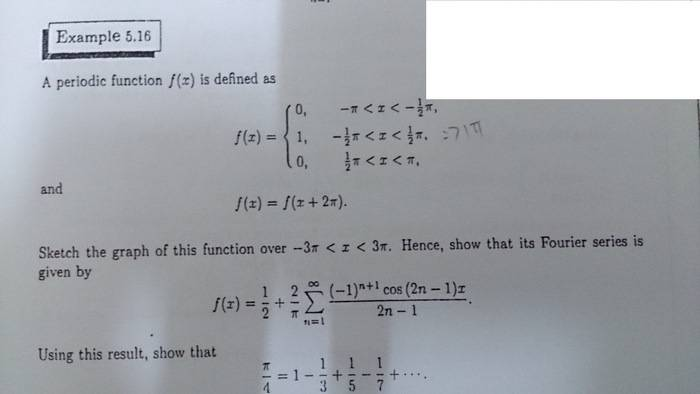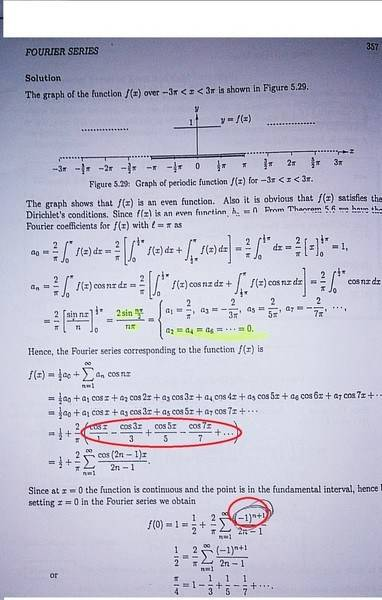# Series to represent alternate between 1 and -1

• foo9008

## Homework Statement

we know that a2 , a4 ,a6 (even number ) = 0 , but when a1 , a3 , a5 (odd numbers) , the answer of an alternate between positive and negative ... in the second circle , the author represent it with (-1)^(n+1) , i don't think this is correct , this is because when n=3 , an = -2/ 3pi when n=3 , [ (-1) ^(3+1 ) ] = positive 1 , not negative 1 ! can someone explain on this ?## The Attempt at a Solution

I think that the author redefined $n$. To make it clear, you start off assuming that

$f(x) = a_0 + \sum_n a_n cos(nx)$

Then, for this particular problem, you find that for $n > 0$, then $a_n = 0$ unless $n$ is odd. If $n$ is odd, then that means that $n$ can be written as:

$n = 2n'-1$

where $n' = 1, 2, 3, ...$

So the term $-\frac{2}{\pi} \frac{cos(3x)}{1}$ corresponds to $n=3$, but it corresponds to $n' = 2$. In terms of $n'$, the general term is

$\frac{2}{\pi} \frac{cos((2n'-1)x)}{2n'-1} (-1)^{n'+1}$